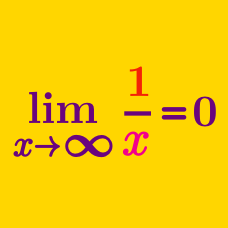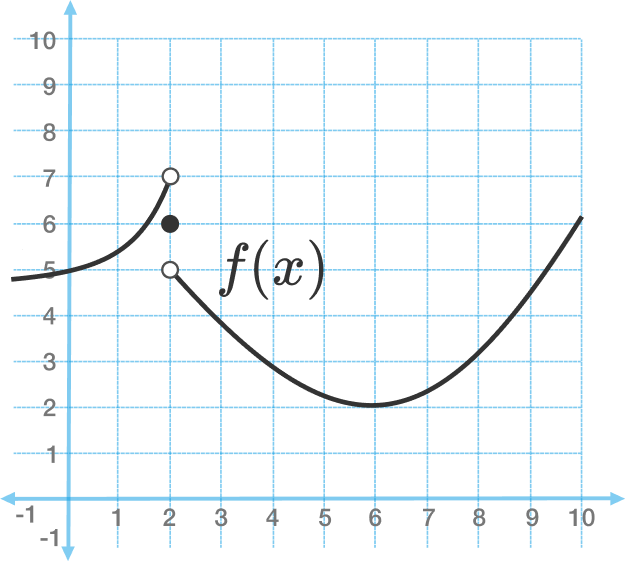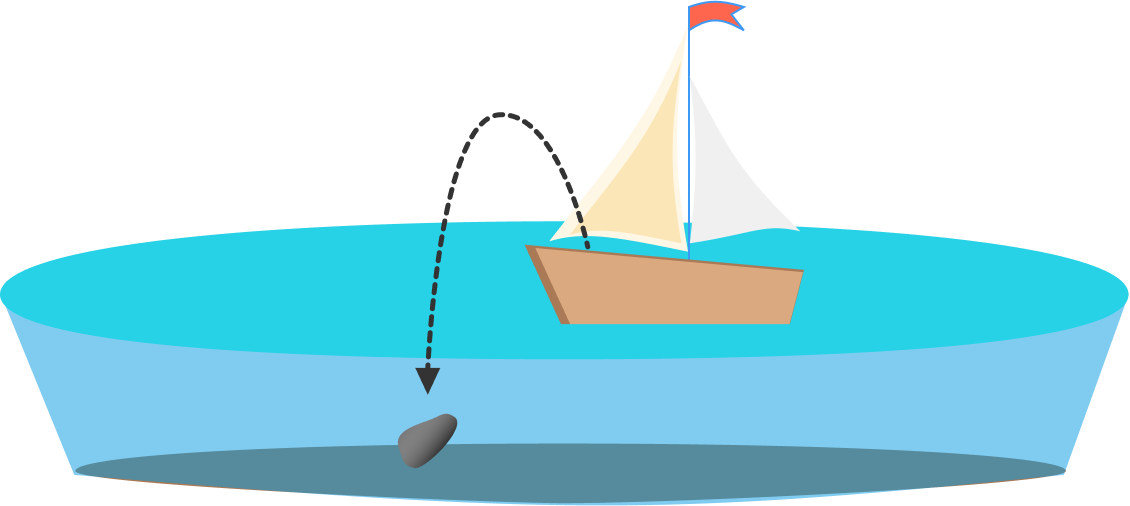Calculus

# Limits of Functions: Level 3 ChallengesFrom the graph of $f$, evaluate

$\Large \lim_{x\to6}f(f(x)).$

Some people claim that $0 ^ 0 = 1$. What is

$\Large \lim_{ x \rightarrow 0^+ } x ^{^ { \frac{ 1}{\ln x} }}?$A man stuck in a small sailboat on a perfectly calm lake throws a stone overboard. It sinks to the bottom of the lake.

When the water again settles to a perfect calm, is the water level in the lake higher, lower, or in the same place compared to where it was before the stone was cast in?

Hint: You can use limits to solve this problem!

Evaluate

$\lim_{x \rightarrow 0^+ } \sqrt{ x + \sqrt{ x + \sqrt{ x + \ldots } } }.$

$\large \lim_{x \to 1} \left( \frac{23}{1-x^{23}}-\frac{11}{1-x^{11}} \right) = \, ?$

×